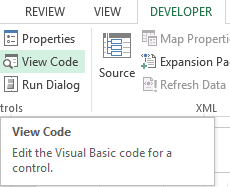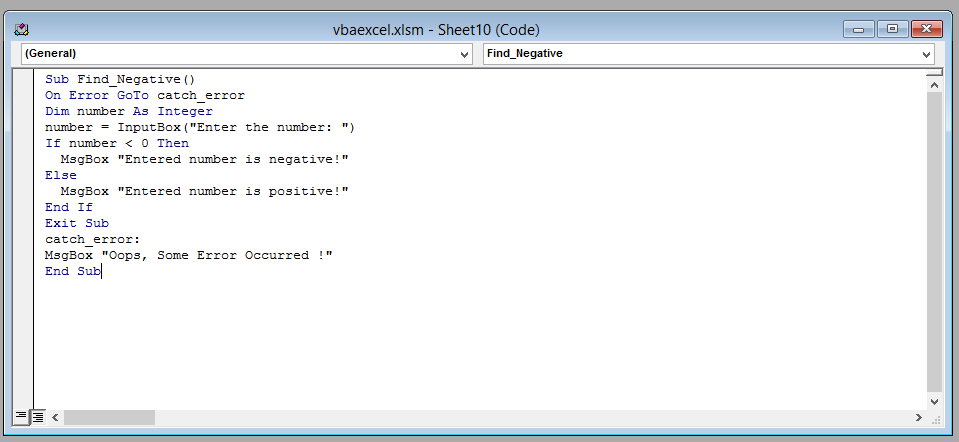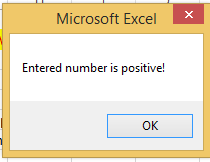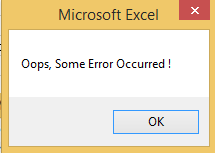### IF Then In Excel

In this article you are going to focus on the If statement. The If statement is a condition.

Conditions are very useful in programming because they allow the user to execute actions based on specific criteria (that the user defines). IF is one of the most widely used and popular statement in excel VBA. The If statement is also sometimes referred to as the If else statement. The purpose of the If statement is to check whether the condition (or conditions) defined by the user are met or not.

This is the syntax of the If statement:

```IF condition1 THEN
'What will the happen if the condition is met
ELSE
'What will the happen if the condition is not met
END IF
```

Explanation: The user defines the condition after the if statement to check whether it’s true or false. If the condition is true than the user in the next line defines what the code will do. However if the condition are not met then the user uses the ELSE statement after which he/she defines what will happen if the condition are not met.

We are going to learn how to use the If statement by making a program in Excel VBA. Our program will take an input from the user and tell whether it’s a positive number or a negative number

Click on Developer tab and select “View Code“.A new window (Visual Basic Editor) will open which would have a dialog box in the center. You will write the code in the dialog box.Write the following line of code in the dialog box.

```Sub Find_Even_Odd()
On Error GoTo catch_error
Dim number As Integer
number = InputBox("Enter the number: ")
If number Mod 2 = 0 Then
MsgBox "Entered number is Even!"
Else
MsgBox "Entered number is Odd!"
End If
Exit Sub
catch_error:
MsgBox "Oops, Some Error Occurred"
End Sub
```

After writing the code close the window by clicking on the cross(x) icon on the upper right side of the screen.

Explanation: In this code, we are first taking the input from the user (the input must be a number).we are checking whether the modulus (Mod) of that number with 2 is zero or not. If its zero than this means that the number is divisible by 2 and thus we display a message box saying the number is positive. If its modulus with 2 is not zero than that number is not perfectly divisible by 2. Hence we display a message box saying that the number is negative. However if the user instead of entering a number enters a letter or a special character (like @) than we display a message box saying that an “error has occurred”.This is the result if the user has entered a positive number.This is the result if the user has entered a negative number.This is the result if the user has entered a special character or a letter.That’s it you have now successfully used the If statement.

## Template

```Further reading: